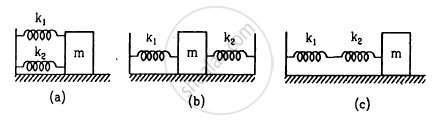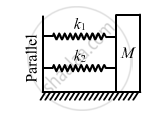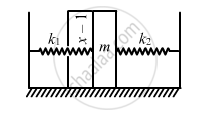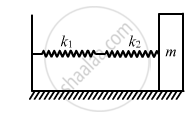Department of Pre-University Education, KarnatakaPUC Karnataka Science Class 12
Advertisement Remove all ads

# Find the Time Period of the Oscillation of Mass M in Figures 12−E4 A, B, C. What is the Equivalent Spring Constant of the Pair of Springs in Each Case? - Physics

Sum

Find the time period of the oscillation of mass m in figures  a, b, c. What is the equivalent spring constant of the pair of springs in each case?]Advertisement Remove all ads

#### Solution

(a) Spring constant of a parallel combination of springs is given as,
K = k1 + k2  (parallel)
Using the relation of time period for S.H.M. for the given spring-mass system, we have :

$T = 2\pi\sqrt{\frac{m}{K}} = 2\pi\sqrt{\frac{m}{k_1 + k_2}}$(b) Let be the displacement of the block of mass m, towards left.
Resultant force is calculated as,
F = F1 + F2 = (k1 + k2)x

Acceleration $\left( a \right)$ is given by,

$a = \left( \frac{F}{m} \right) = \frac{\left( k_1 + k_2 \right)}{m}x$

Time period $\left( T \right)$ is given by ,

$T = 2\pi\sqrt{\frac{\text { displacement }}{\text { acceleration }}}$

$\text { On substituting the values of displacement and acceleration, we get: }$ $T = 2\pi\sqrt{\frac{x}{x\frac{\left( k_1 + k_2 \right)}{m}}}$

$= 2\pi\sqrt{\frac{m}{k_1 + k_2}}$Required spring constant, K = k1 + k2

(c) Let K be the equivalent spring constant of the series combination.

$\frac{1}{K} = \frac{1}{k_1} + \frac{1}{k_2} = \frac{k_2 + k_1}{k_1 k_2}$

$\Rightarrow K = \frac{k_1 k_2}{k_1 + k_2}$

$\text { Time period is given by, }$

$T = 2\pi\sqrt{\frac{m}{K}}$

$\text { On substituting the respective values, we get: }$ $T = 2\pi\sqrt{\frac{m\left( k_1 + k_2 \right)}{k_1 k_2}}$Is there an error in this question or solution?
Advertisement Remove all ads

#### APPEARS IN

HC Verma Class 11, 12 Concepts of Physics 1
Chapter 12 Simple Harmonics Motion
Q 17 | Page 253
Advertisement Remove all ads

#### Video TutorialsVIEW ALL 

Advertisement Remove all ads
Share
Notifications

View all notifications

Forgot password?